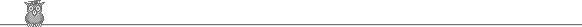# Equilibrium

Name

In the Land of Hialeah the supply curve for roses is given by

Q = -10 + 1.5*P

and the demand curve for roses is given by

Q = 10 - 0.5 P

A. When the quantity demanded is 5 what must have been the price?

B. When the price is 15 what is the quantity supplied?

C. What are the equilibrium price and quantity? P Q

Consumer surplus is defined as the difference between what consumers are willing to pay (points on the demand curve) and the price that they have to pay, aggregated over all consumers.  Graphically, it is the triangle area under the demand curve but above the market price.

D. Given the equilibrium price and quantity, what is consumer surplus in the market for roes?

E. Suppose that a \$.50 excise tax (i.e. a per unit tax) is collected from the supplier.  If the seller is to continue supplying the same quantity as at the 'no tax' equilibrium what gross price must she receive?

F. What will be the new equilibrium price and quantity? P  Q

G. What is the change in price paid by the consumer between parts C. and F. (before and after the imposition of the tax)?

H. What is the change in the price received by the seller, net of taxes?

I. As a result of the tax does consumer surplus go up or down ?